Скачать презентацию Chapter 7 Outline Bonds and Bond

• Количество слайдов: 34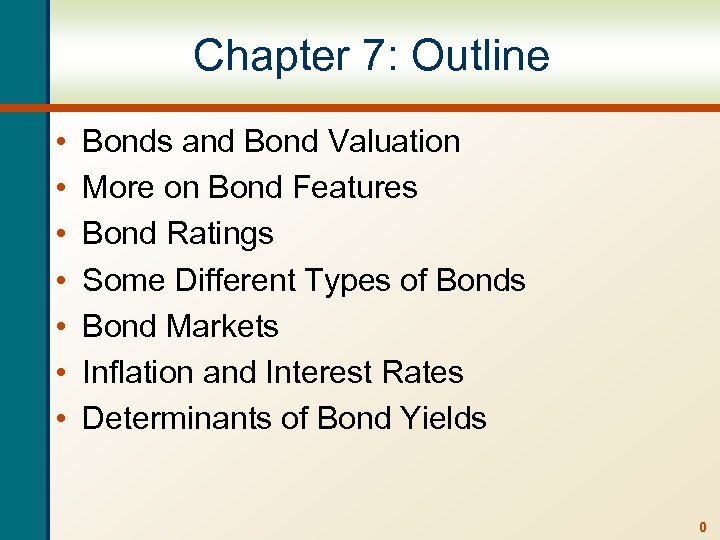Chapter 7: Outline • • Bonds and Bond Valuation More on Bond Features Bond Ratings Some Different Types of Bonds Bond Markets Inflation and Interest Rates Determinants of Bond Yields 0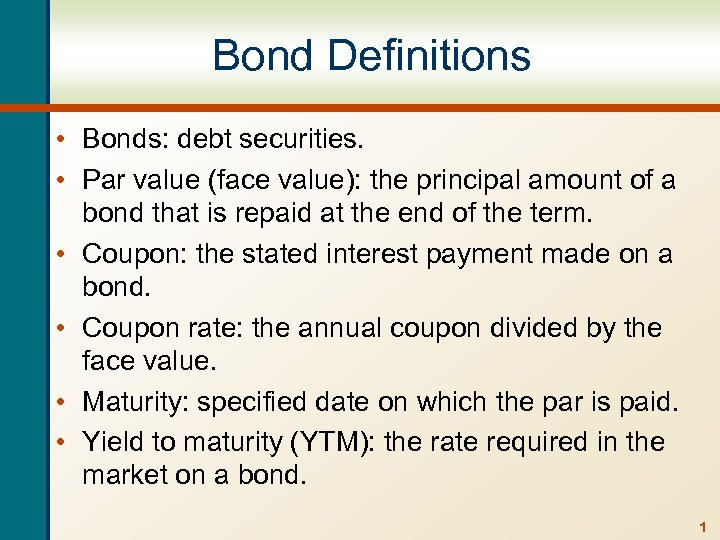Bond Definitions • Bonds: debt securities. • Par value (face value): the principal amount of a bond that is repaid at the end of the term. • Coupon: the stated interest payment made on a bond. • Coupon rate: the annual coupon divided by the face value. • Maturity: specified date on which the par is paid. • Yield to maturity (YTM): the rate required in the market on a bond. 1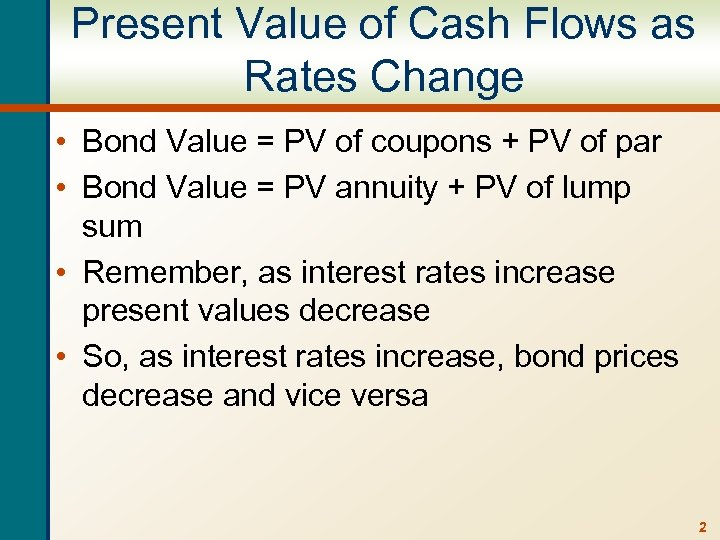Present Value of Cash Flows as Rates Change • Bond Value = PV of coupons + PV of par • Bond Value = PV annuity + PV of lump sum • Remember, as interest rates increase present values decrease • So, as interest rates increase, bond prices decrease and vice versa 2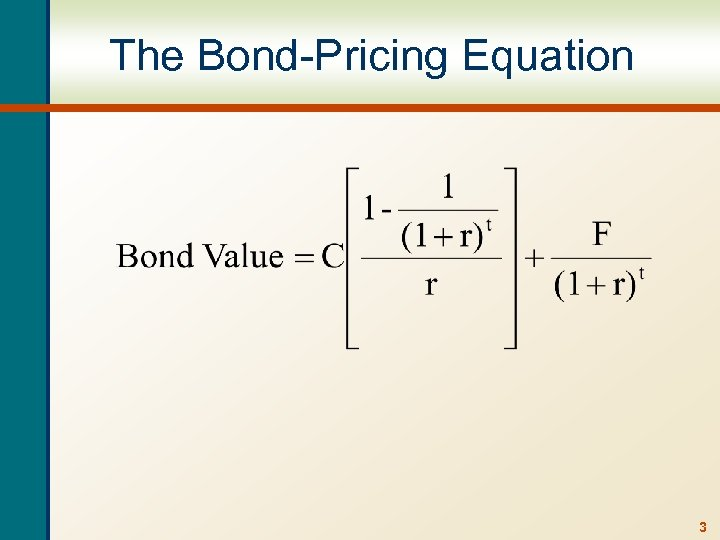The Bond-Pricing Equation 3Valuing a Bond with Annual Coupons • Consider a bond with a coupon rate of 10% and annual coupons. The par value is \$1000 and the bond has 5 years to maturity. The yield to maturity is 11%. What is the value of the bond? • Using the formula: • B = PV of annuity + PV of lump sum • B = 100[1 – 1/(1. 11)5] /. 11 + 1000 / (1. 11)5 • B = 369. 59 + 593. 45 = 963. 04 • Using the calculator: • N = 5; I/Y = 11; PMT = 100; FV = 1000 • CPT PV = -963. 04 4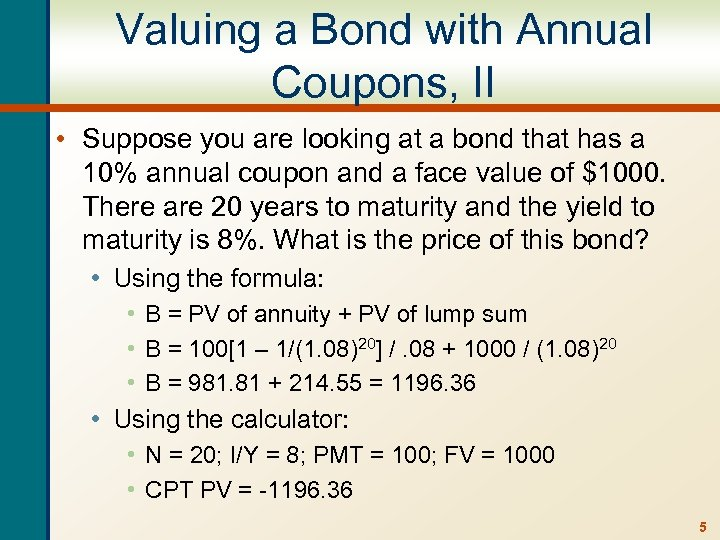Valuing a Bond with Annual Coupons, II • Suppose you are looking at a bond that has a 10% annual coupon and a face value of \$1000. There are 20 years to maturity and the yield to maturity is 8%. What is the price of this bond? • Using the formula: • B = PV of annuity + PV of lump sum • B = 100[1 – 1/(1. 08)20] /. 08 + 1000 / (1. 08)20 • B = 981. 81 + 214. 55 = 1196. 36 • Using the calculator: • N = 20; I/Y = 8; PMT = 100; FV = 1000 • CPT PV = -1196. 36 5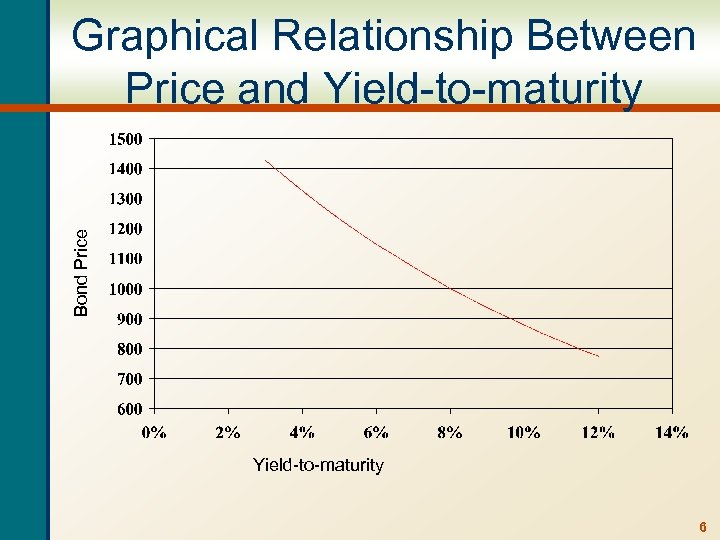Bond Price Graphical Relationship Between Price and Yield-to-maturity 6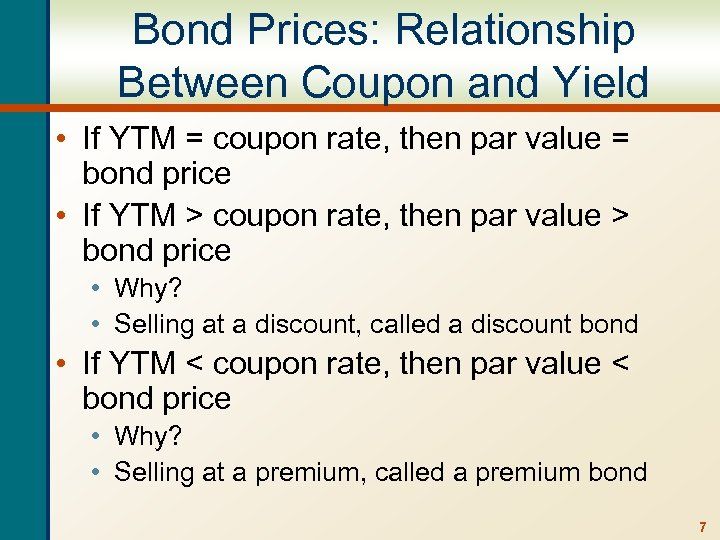Bond Prices: Relationship Between Coupon and Yield • If YTM = coupon rate, then par value = bond price • If YTM > coupon rate, then par value > bond price • Why? • Selling at a discount, called a discount bond • If YTM < coupon rate, then par value < bond price • Why? • Selling at a premium, called a premium bond 7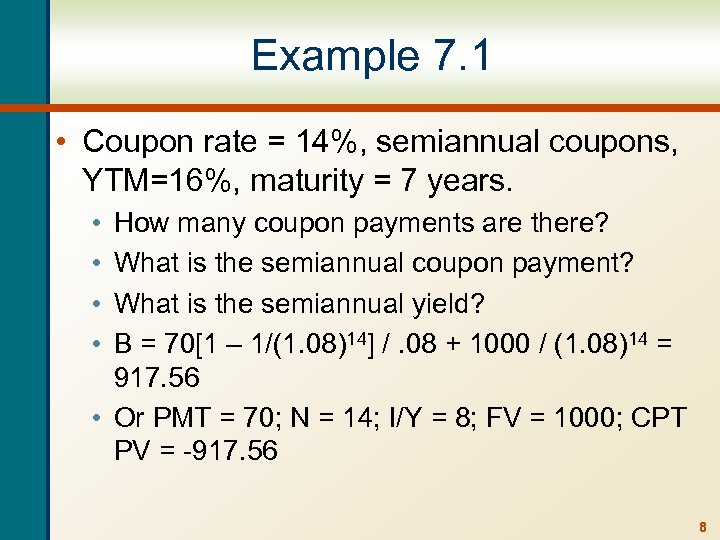Example 7. 1 • Coupon rate = 14%, semiannual coupons, YTM=16%, maturity = 7 years. • • How many coupon payments are there? What is the semiannual coupon payment? What is the semiannual yield? B = 70[1 – 1/(1. 08)14] /. 08 + 1000 / (1. 08)14 = 917. 56 • Or PMT = 70; N = 14; I/Y = 8; FV = 1000; CPT PV = -917. 56 8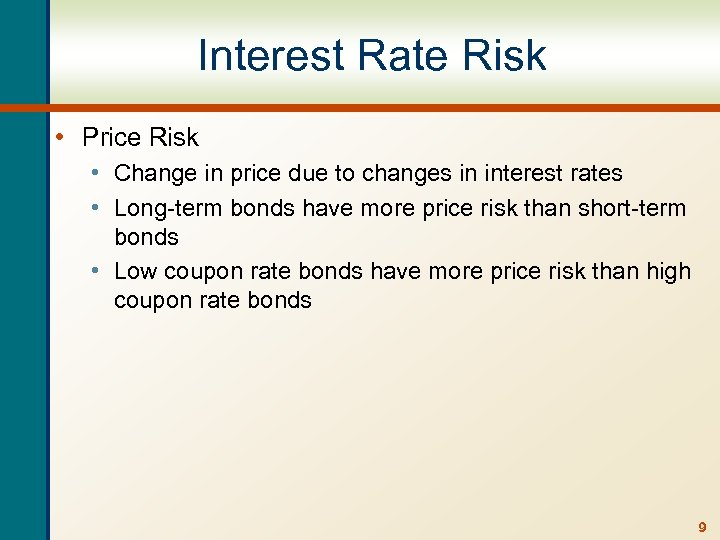Interest Rate Risk • Price Risk • Change in price due to changes in interest rates • Long-term bonds have more price risk than short-term bonds • Low coupon rate bonds have more price risk than high coupon rate bonds 9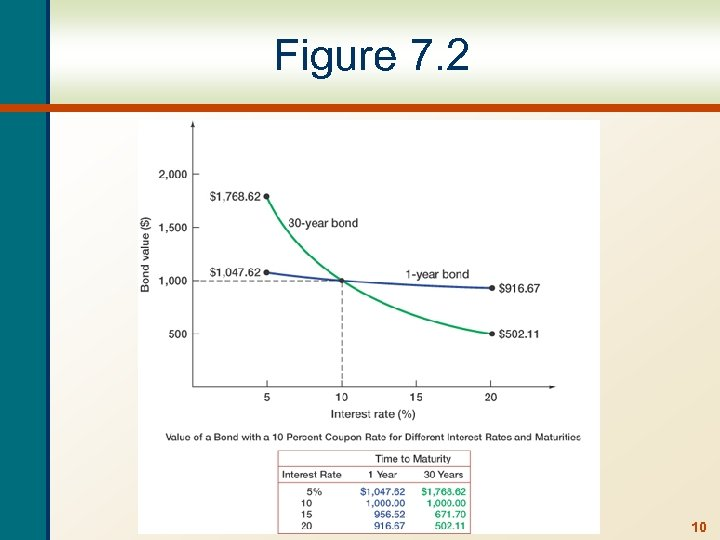Figure 7. 2 10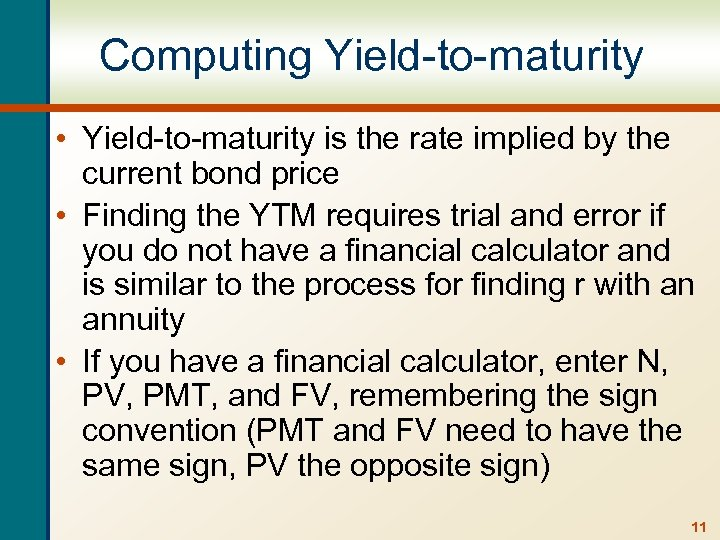Computing Yield-to-maturity • Yield-to-maturity is the rate implied by the current bond price • Finding the YTM requires trial and error if you do not have a financial calculator and is similar to the process for finding r with an annuity • If you have a financial calculator, enter N, PV, PMT, and FV, remembering the sign convention (PMT and FV need to have the same sign, PV the opposite sign) 11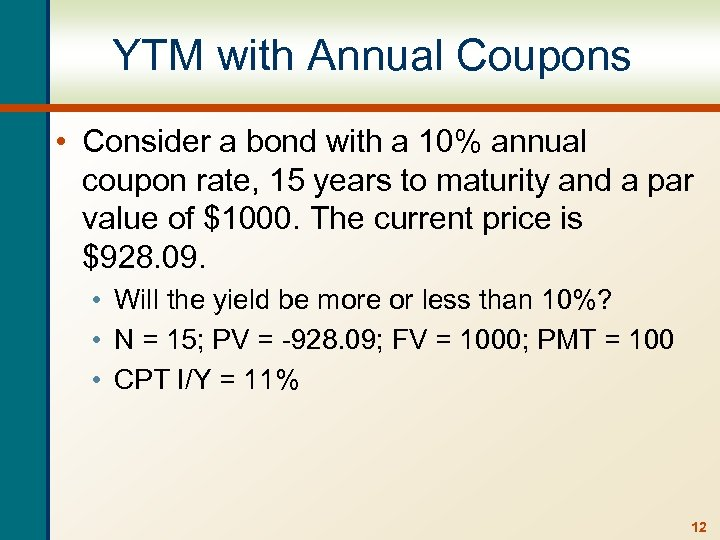YTM with Annual Coupons • Consider a bond with a 10% annual coupon rate, 15 years to maturity and a par value of \$1000. The current price is \$928. 09. • Will the yield be more or less than 10%? • N = 15; PV = -928. 09; FV = 1000; PMT = 100 • CPT I/Y = 11% 12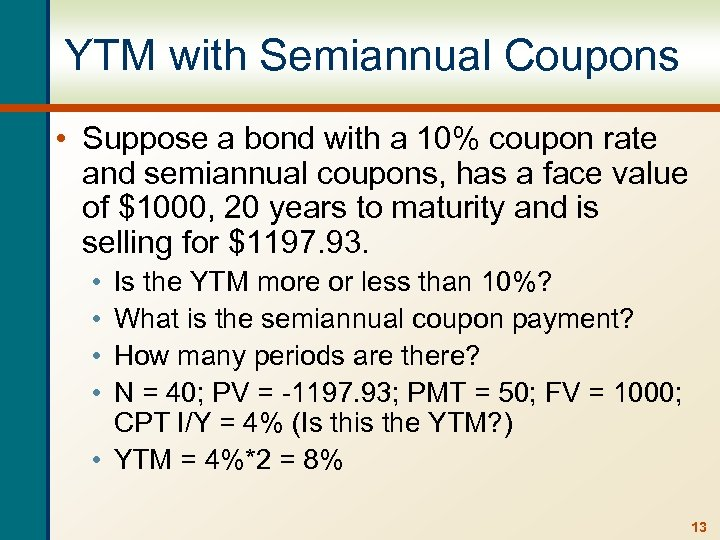YTM with Semiannual Coupons • Suppose a bond with a 10% coupon rate and semiannual coupons, has a face value of \$1000, 20 years to maturity and is selling for \$1197. 93. • • Is the YTM more or less than 10%? What is the semiannual coupon payment? How many periods are there? N = 40; PV = -1197. 93; PMT = 50; FV = 1000; CPT I/Y = 4% (Is this the YTM? ) • YTM = 4%*2 = 8% 13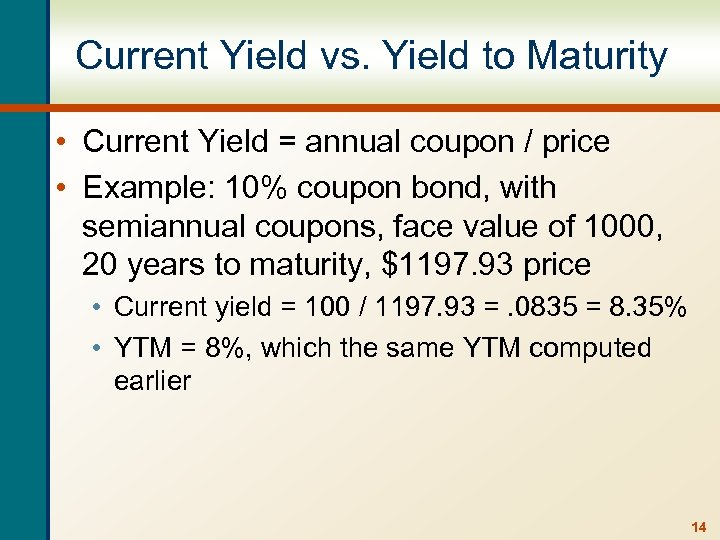Current Yield vs. Yield to Maturity • Current Yield = annual coupon / price • Example: 10% coupon bond, with semiannual coupons, face value of 1000, 20 years to maturity, \$1197. 93 price • Current yield = 100 / 1197. 93 =. 0835 = 8. 35% • YTM = 8%, which the same YTM computed earlier 14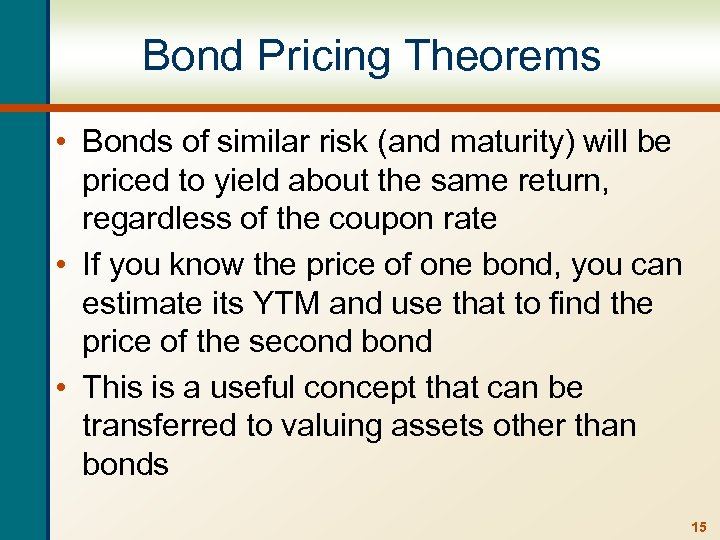Bond Pricing Theorems • Bonds of similar risk (and maturity) will be priced to yield about the same return, regardless of the coupon rate • If you know the price of one bond, you can estimate its YTM and use that to find the price of the second bond • This is a useful concept that can be transferred to valuing assets other than bonds 15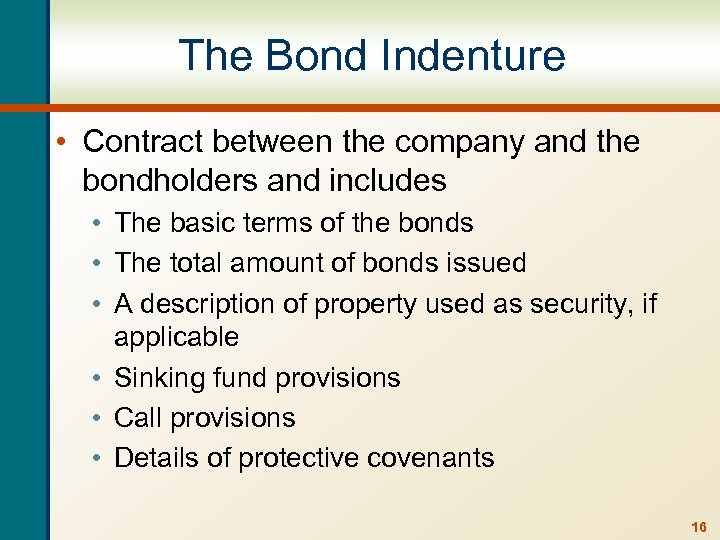The Bond Indenture • Contract between the company and the bondholders and includes • The basic terms of the bonds • The total amount of bonds issued • A description of property used as security, if applicable • Sinking fund provisions • Call provisions • Details of protective covenants 16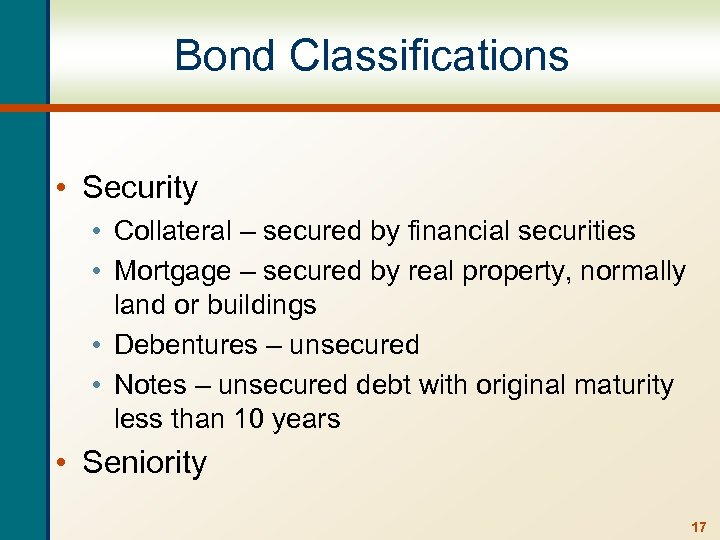Bond Classifications • Security • Collateral – secured by financial securities • Mortgage – secured by real property, normally land or buildings • Debentures – unsecured • Notes – unsecured debt with original maturity less than 10 years • Seniority 17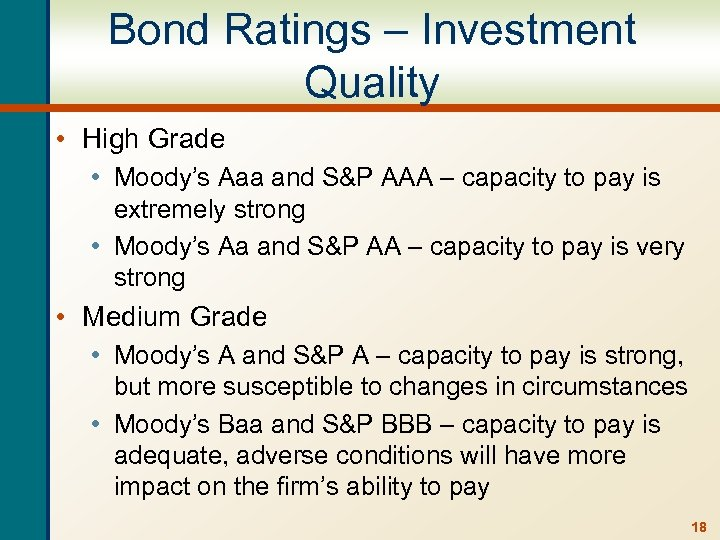Bond Ratings – Investment Quality • High Grade • Moody’s Aaa and S&P AAA – capacity to pay is extremely strong • Moody’s Aa and S&P AA – capacity to pay is very strong • Medium Grade • Moody’s A and S&P A – capacity to pay is strong, but more susceptible to changes in circumstances • Moody’s Baa and S&P BBB – capacity to pay is adequate, adverse conditions will have more impact on the firm’s ability to pay 18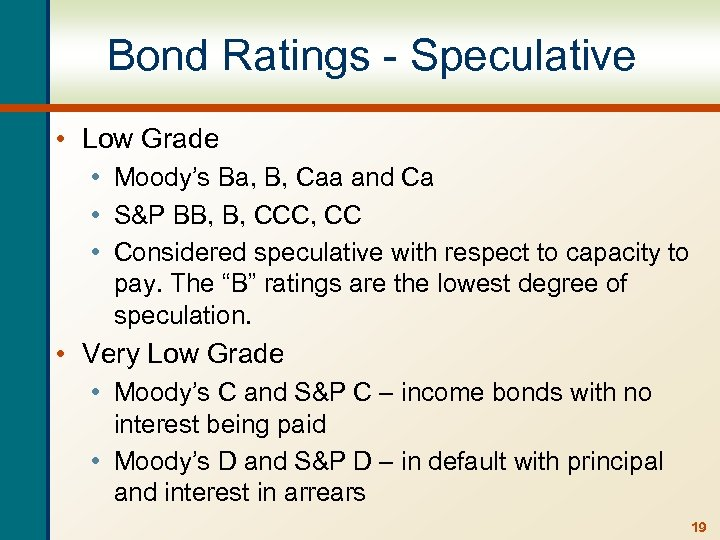Bond Ratings - Speculative • Low Grade • Moody’s Ba, B, Caa and Ca • S&P BB, B, CCC, CC • Considered speculative with respect to capacity to pay. The “B” ratings are the lowest degree of speculation. • Very Low Grade • Moody’s C and S&P C – income bonds with no interest being paid • Moody’s D and S&P D – in default with principal and interest in arrears 19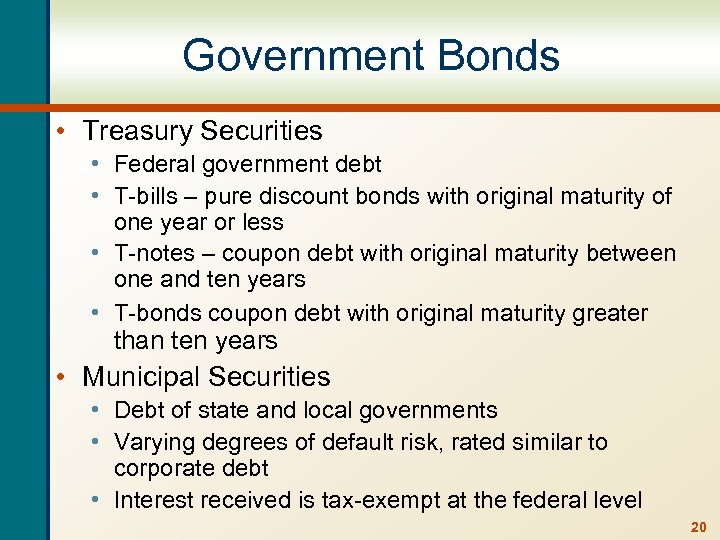Government Bonds • Treasury Securities • Federal government debt • T-bills – pure discount bonds with original maturity of one year or less • T-notes – coupon debt with original maturity between one and ten years • T-bonds coupon debt with original maturity greater than ten years • Municipal Securities • Debt of state and local governments • Varying degrees of default risk, rated similar to corporate debt • Interest received is tax-exempt at the federal level 20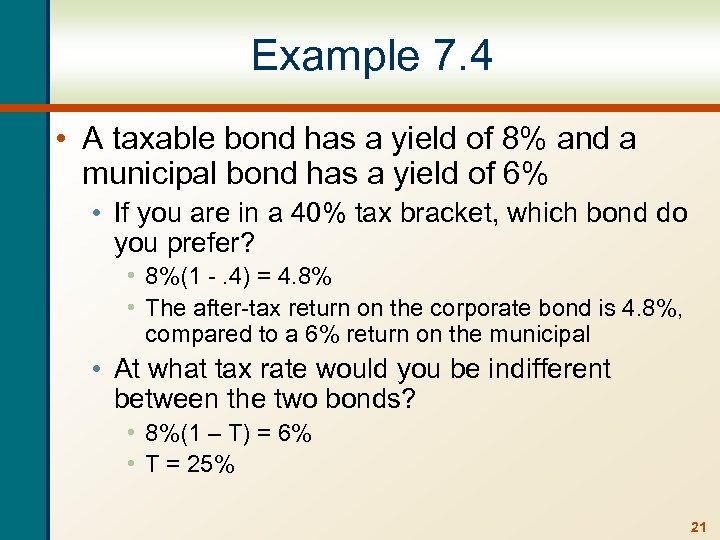Example 7. 4 • A taxable bond has a yield of 8% and a municipal bond has a yield of 6% • If you are in a 40% tax bracket, which bond do you prefer? • 8%(1 -. 4) = 4. 8% • The after-tax return on the corporate bond is 4. 8%, compared to a 6% return on the municipal • At what tax rate would you be indifferent between the two bonds? • 8%(1 – T) = 6% • T = 25% 21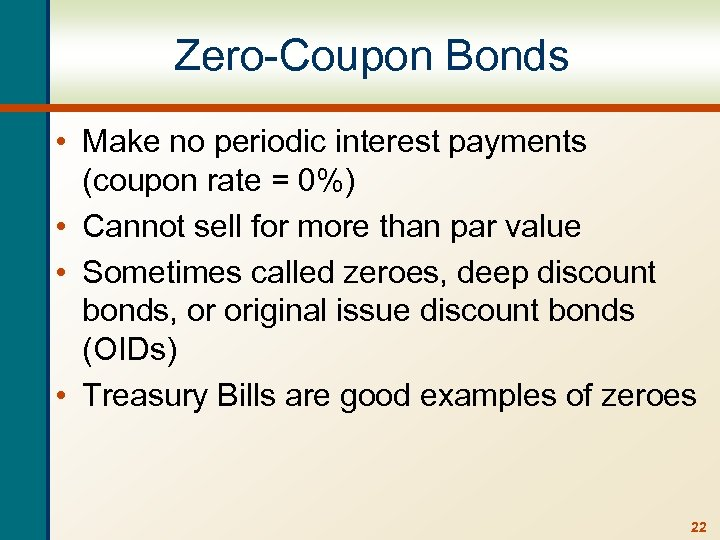Zero-Coupon Bonds • Make no periodic interest payments (coupon rate = 0%) • Cannot sell for more than par value • Sometimes called zeroes, deep discount bonds, or original issue discount bonds (OIDs) • Treasury Bills are good examples of zeroes 22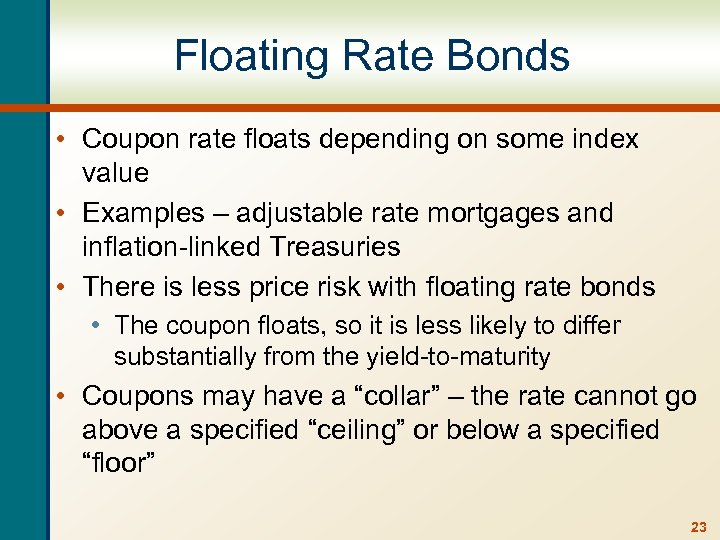Floating Rate Bonds • Coupon rate floats depending on some index value • Examples – adjustable rate mortgages and inflation-linked Treasuries • There is less price risk with floating rate bonds • The coupon floats, so it is less likely to differ substantially from the yield-to-maturity • Coupons may have a “collar” – the rate cannot go above a specified “ceiling” or below a specified “floor” 23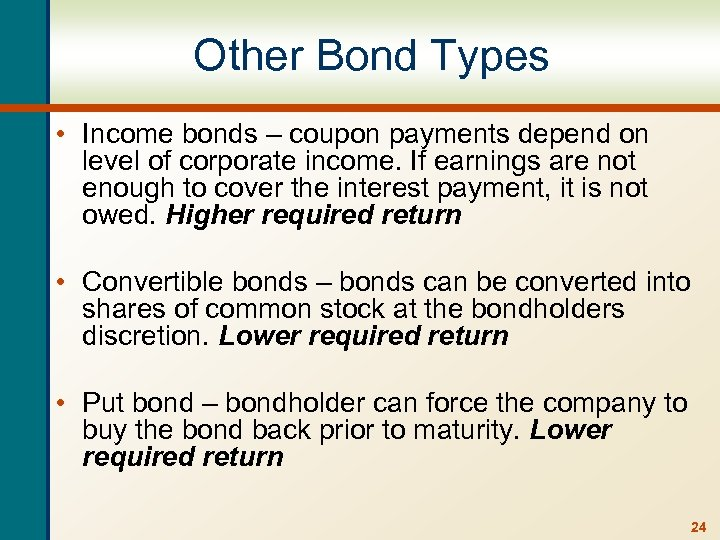Other Bond Types • Income bonds – coupon payments depend on level of corporate income. If earnings are not enough to cover the interest payment, it is not owed. Higher required return • Convertible bonds – bonds can be converted into shares of common stock at the bondholders discretion. Lower required return • Put bond – bondholder can force the company to buy the bond back prior to maturity. Lower required return 24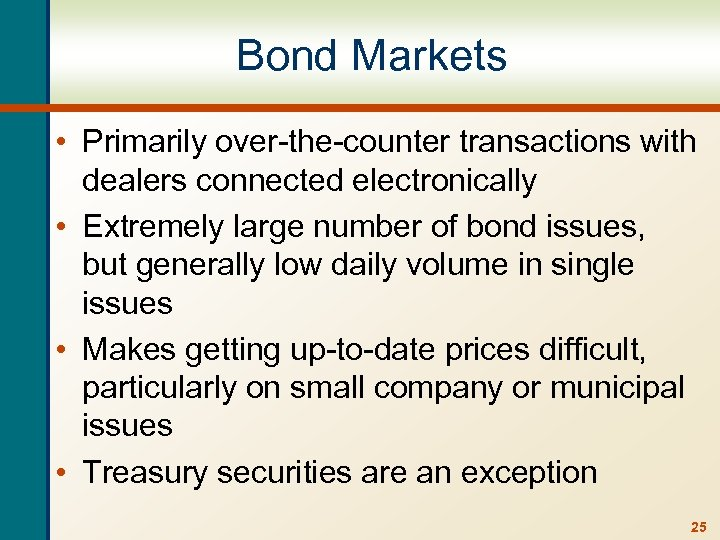Bond Markets • Primarily over-the-counter transactions with dealers connected electronically • Extremely large number of bond issues, but generally low daily volume in single issues • Makes getting up-to-date prices difficult, particularly on small company or municipal issues • Treasury securities are an exception 25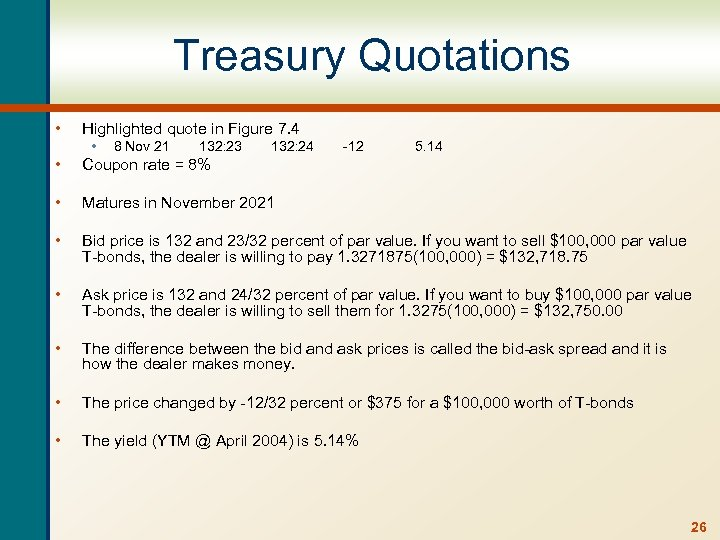Treasury Quotations • Highlighted quote in Figure 7. 4 • 8 Nov 21 132: 23 132: 24 -12 5. 14 • Coupon rate = 8% • Matures in November 2021 • Bid price is 132 and 23/32 percent of par value. If you want to sell \$100, 000 par value T-bonds, the dealer is willing to pay 1. 3271875(100, 000) = \$132, 718. 75 • Ask price is 132 and 24/32 percent of par value. If you want to buy \$100, 000 par value T-bonds, the dealer is willing to sell them for 1. 3275(100, 000) = \$132, 750. 00 • The difference between the bid and ask prices is called the bid-ask spread and it is how the dealer makes money. • The price changed by -12/32 percent or \$375 for a \$100, 000 worth of T-bonds • The yield (YTM @ April 2004) is 5. 14% 26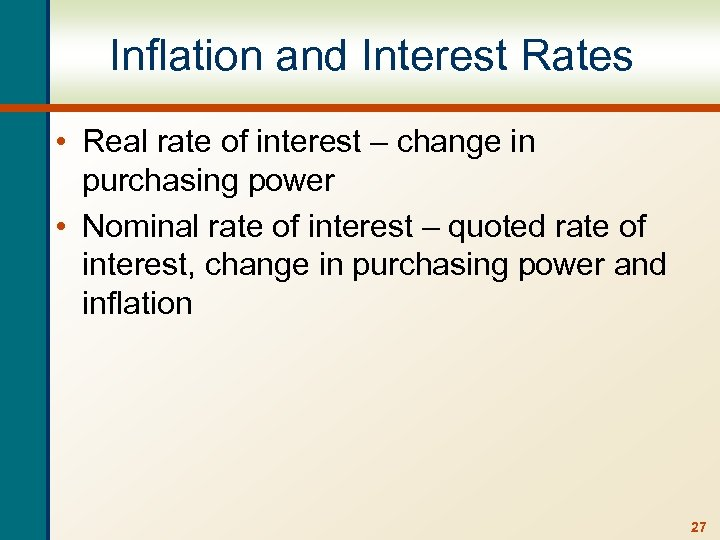Inflation and Interest Rates • Real rate of interest – change in purchasing power • Nominal rate of interest – quoted rate of interest, change in purchasing power and inflation 27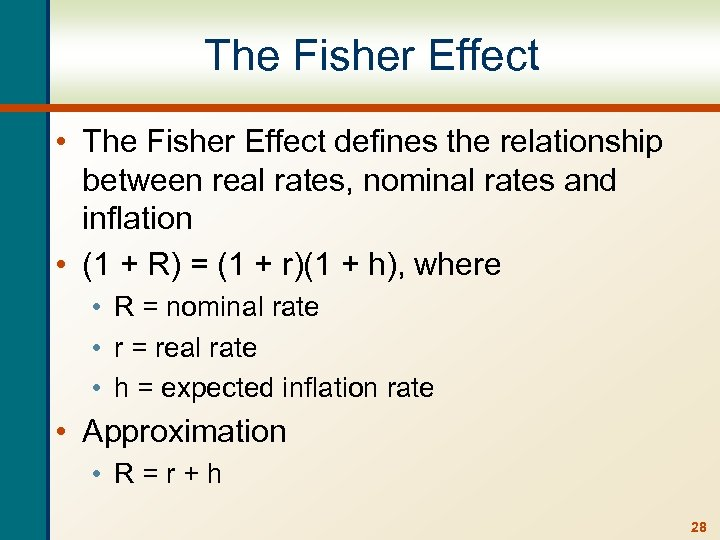The Fisher Effect • The Fisher Effect defines the relationship between real rates, nominal rates and inflation • (1 + R) = (1 + r)(1 + h), where • R = nominal rate • r = real rate • h = expected inflation rate • Approximation • R=r+h 28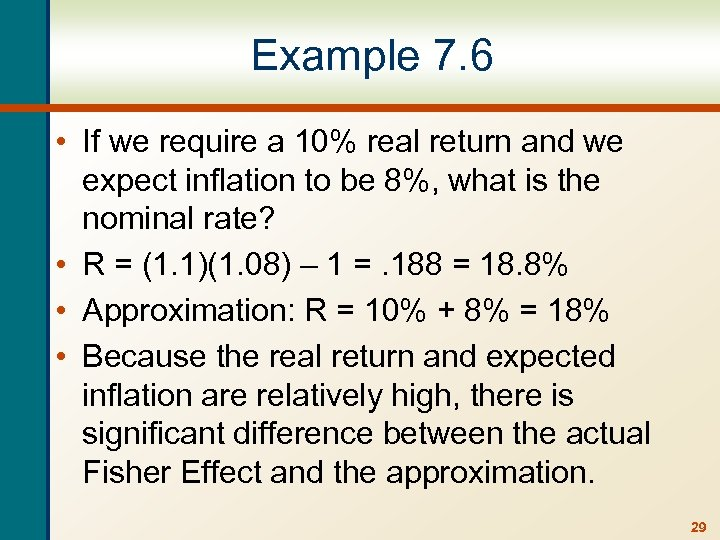Example 7. 6 • If we require a 10% real return and we expect inflation to be 8%, what is the nominal rate? • R = (1. 1)(1. 08) – 1 =. 188 = 18. 8% • Approximation: R = 10% + 8% = 18% • Because the real return and expected inflation are relatively high, there is significant difference between the actual Fisher Effect and the approximation. 29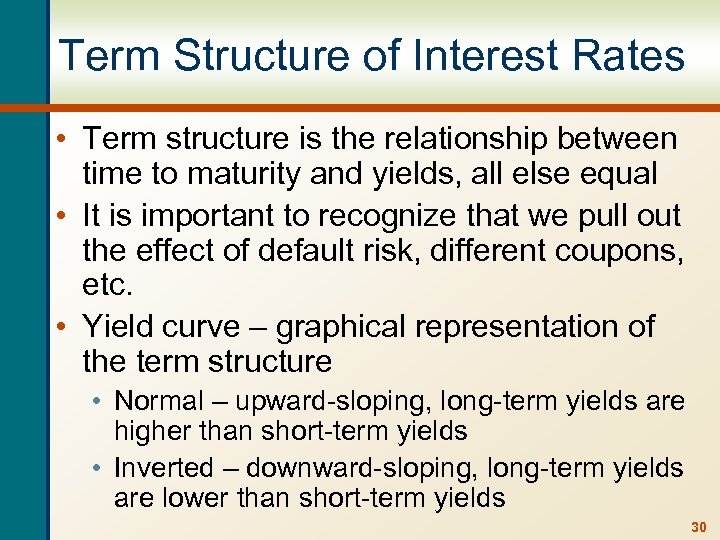Term Structure of Interest Rates • Term structure is the relationship between time to maturity and yields, all else equal • It is important to recognize that we pull out the effect of default risk, different coupons, etc. • Yield curve – graphical representation of the term structure • Normal – upward-sloping, long-term yields are higher than short-term yields • Inverted – downward-sloping, long-term yields are lower than short-term yields 30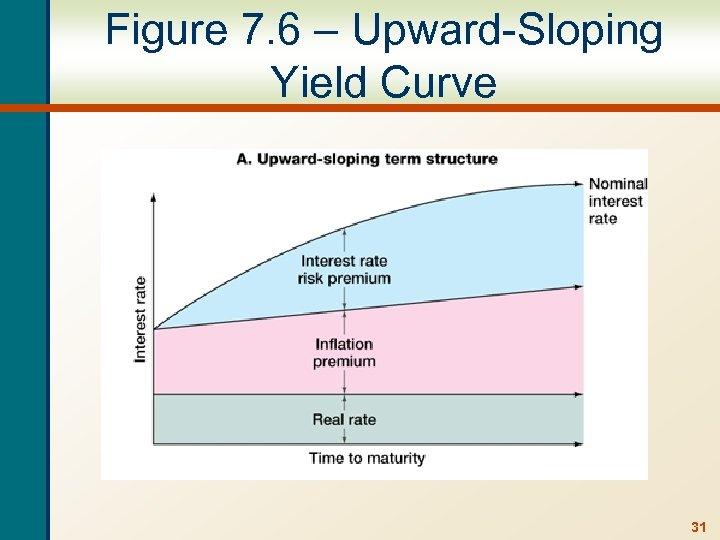Figure 7. 6 – Upward-Sloping Yield Curve 31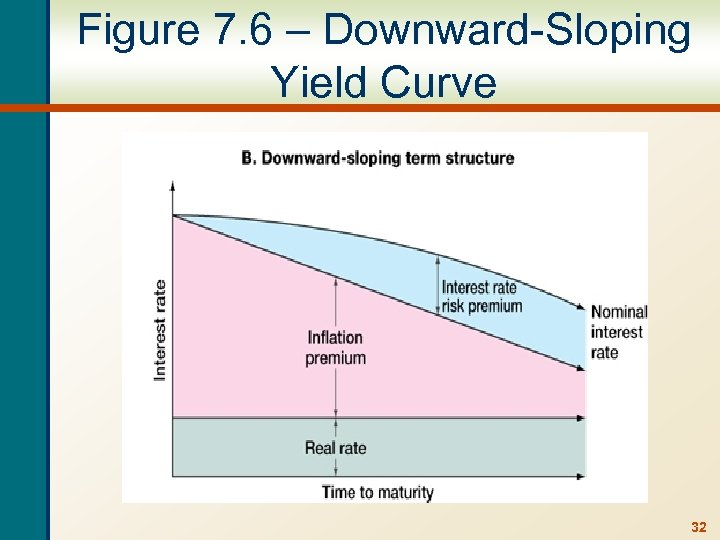Figure 7. 6 – Downward-Sloping Yield Curve 32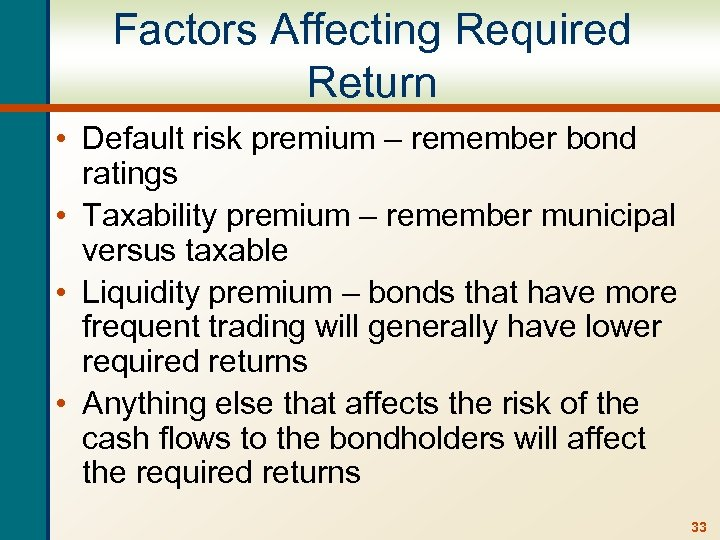Factors Affecting Required Return • Default risk premium – remember bond ratings • Taxability premium – remember municipal versus taxable • Liquidity premium – bonds that have more frequent trading will generally have lower required returns • Anything else that affects the risk of the cash flows to the bondholders will affect the required returns 33